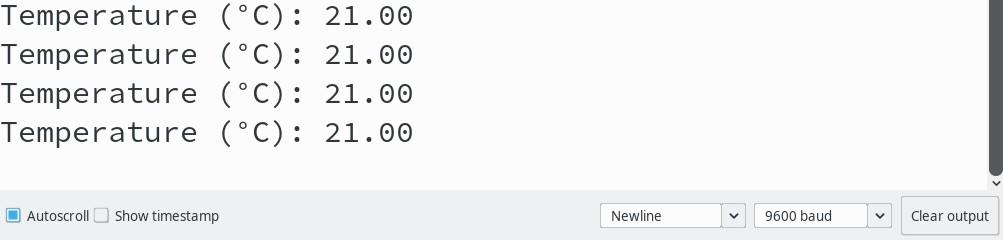# LM35 Temperature Sensor

The LM35 is a cheap and easy to use analog temperature sensor found in many Arduino Kits. Let's find out how to use it.

## LM35 Temperature Sensor

The LM35 is a factory calibrated silicon band gap temperature sensor manufactured by Texas Instruments. It makes use of the fact that the forward voltage of the p-n-junction in diodes or transistors is temperature dependent. The fact that the sensing element does not require any special materials to be integrated in to the die makes this type of temperature sensors easy to integrate and cheaper to produce. However, different from the DS18B20 which is a band gap temperature sensor as well, the LM35 has an analog output instead of a digital one. It signals the temperature in degree Celsius via the output voltage at the Vout pin.

For the LM35DZ, that we are going to use here, an output voltage of 0 V corresponds to 0° C. The output voltage increases by 10 mV/°C. Obviously, this sensor is specifically designed for a temperature output in degree Celsius. For an output in degree Fahrenheit you should use the LM34 instead. The LM35DZ can measure temperatures up to 100 °C which corresponds to an output voltage of 1 V. To get the current temperature, we just need to measure the sensors output voltage with one of the Arduino's analog inputs. Let's see how that works!

## Wiring Up the Sensor

Wiring up the sensor is no big deal. With the flat side facing up, the first pin is for the supply voltage, the second is the analog output and the third one is the ground pin. Simply connect the sensor as shown in the image below, with its analog output connected to A0 and its supply voltage pin connected to the Arduino's 5V pin:## Writing the Code

Let's have a look at the code:

void setup() {
// Initialize serial communication
Serial.begin(9600);
}

void loop() {
float voltage = analogRead(A0) * 5.0 / 1024.0;

// Temperature: 10 mV/°C
float temperature = voltage / 0.01;

// Output to serial monitor
Serial.print("Temperature (°C): ");
Serial.println(temperature);
delay(500);
}

The program uses the analogRead function to measure the voltage at A0. The Arduino's analog-to-digital converter (ADC) converts the analog voltage into a number between 0 and 1023. A value of 1024 would be equivalent to the reference voltage of the ADC which by default corresponds to the Arduino's supply voltage of 5 V. To convert it into a voltage, we can use the following formula:

$$V_{A0} = {V_{Ref} \cdot analogRead(A0) \over 1024}$$

This is exactly what is done in the code. After that the voltage can be easily converted into a temperature. As 10 mV correspond 1 °C we simply divide by 10 mV/°C and get the temperature in degree Celsius. That's all there is to do.

After downloading the program onto the Arduino, the temperature is displayed in the serial monitor every 500 ms:## Improving the Accuracy

The LM35 typically has an accuracy of ± 0.6 °C at 25 °C. We can't change that. However, we can improve the accuracy of the ADC readings done by the Arduino Uno. The output voltage of the sensor is between 0 V and 1 V while the Arduino Uno gives us a voltage reading between 0 V and 5 V in 1024 steps. This means we are left with only 204 steps between 0 V and 1 V which is equivalent to resolution of 4.9 mV or roughly 0.5 °C. This is not horrific, but sufficient as this corresponds roughly to the sensors accuracy. However, a problem that often occurs when measuring analog voltages with the Arduino Uno is, that the supply voltage is not exactly 5 V. This is especially true if the Arduino is powered via USB and leads to inaccurate measurements as the supply voltage is also used as reference voltage for the ADC.

The Arduino Uno provides us with an alternative that is better suited for our use case. It has an internal 1.1 V voltage reference that is independent of the supply voltage. We can activate it by calling analogReference(INTERNAL) in the setup procedure. Once this voltage reference is activated the ADC measures a voltage between 0 V and 1.1 V instead of 5 V. The measurement is still represented by a number between 0 and 1023. However, the hypothetical value of 1024 now corresponds to 1.1 V instead of 5 V. Using the internal voltage reference, a supply voltage of precisely 5 V is no longer important for an accurate measurement. Additionally, the resolution increases as the measurement range of the ADC (0 V - 1.1 V) corresponds better to the output voltage range of the sensor (0 V - 1 V).

Here is how the adjusted code looks like:

void setup() {
// Initialize serial communication
Serial.begin(9600);

// Use 1.1 V reference for ADC measurements
analogReference(INTERNAL);
}

void loop() {
float voltage = analogRead(A0) * 1.1 / 1024.0;

// Temperature: 10 mV/°C
float temperature = voltage / 0.01;

// Output to serial monitor
Serial.print("Temperature (°C): ");
Serial.println(temperature);
delay(500);
}

## Summing Up

In this tutorial we saw how easy it is to use the LM35. We don't need calibration or complex formulas like with the NTCs, and we don't need a library to interface with a digital sensor via a bus like OneWire or I2C. A single analog pin and a simple voltage measurement is enough to get the temperature.